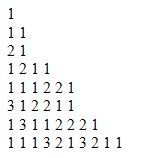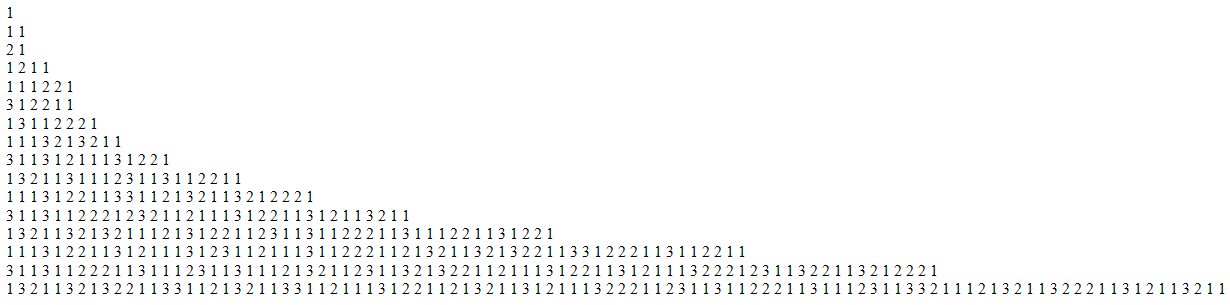﻿ Morris Number Sequence Puzzle Solution in JavascriptjQuery and Javascript Tutorials and Code Examples Javascript Tutorials and Javascript Frameworks jQuery resources, tools, articles, code samples and downloads for Web Programmers

# Number Sequence Puzzle Solution in Javascript

Morris Number Sequence puzzle is one of the popular mathematical puzzles that I want to solve using Javascript for developers to build programming algorithms. Javascript programmers will see that using Array object and Array methods with While and For loops, we can easily solve this problem also know as look-and-say sequence.

Let's first define the problem or question stated in the Morris number sequence puzzle.

Start with 1. As you see in below sequence image 1 is at the top. => 1

The second line can be read as follow: Previous line has one 1. => 1 1

Now, we can try to guess the third line: Previous line (which is line 2) has two 1. => 2 1

Fourth line summarizing the numbers in previous third line will be: One 2 and One 1. => 1 2 1 1

The lines and the content of each line is dependent on previous line and goes on like summarized above.What is important above is that: if same number is on the list side by side we count them. When a different number is in the order we write previously collected data and count the new number starting from 1.

Look and say sequence question is what is the content of the Nth line in this number sequence?

## Javascript Code for Morris Number Sequence

In order to solve this mathematical puzzle (Morris Number Sequence), I preferred to code and simulate the case in Javascript.
I will try to explain the below Javascript code for programmers in order to read it easier.

Lines is the parameter to keep the number of lines to display. Change this if you wonder the sequence numbers for 100th line.

list is Javascript array object to keep number sequence on current line. I populate it with "1" at initialization using Javascript Array.Push() method.

Then a Javascript code block which runs once for each line within Javascript For loop.

At each line's start, I reset parameter current which stores first number in the sequence. And set its count to 0.
Then I read each number in the sequence one by one, if number matches current I increase counter.

If a different number is recognized, I write the counter value and the counted item in summary Javascript array. I use this summary array to store calculations for next line in the sequence.

To store values in summary array, I use Javascript Array.Push() method.

When I reach to the end of that line, since I completed calculations at this point, I can securely get rid of the current line sequence which is stored in Javascript array named list.
Developers can see that I set the length of the list array to 0 in order to truncate Javascript array.
I found this method to delete all items in a Javascript array easier.

After I truncate javascript array list, I copy all items from summary array to list array using Javascript Array.Concat() method.
Array.Concat method adds the input array items to the end of the main array object.

Then I clear array items of summary Javascript array object.

Below is the output of above script with altered input lines parameter for 10 lines of numbers sequence puzzle.

I enjoy solving such well known puzzle in Javascript with simulating the case or problem by coding and hope you, programmers also like this solution of Morris number sequence mathematical puzzle.

If you are interested in Josephus problem and how to solve Josephus problem aka "the last person among 100 people with a sword around a circle", please check referenced Javascript tutorial.

Let's test sample Javascript solution to numbers sequence puzzle for 20th line.Javascript